This geom is used to visualise SpatRaster objects (see terra::rast()). The geom is designed for visualise the object by layers, as terra::plot() does.

For plotting SpatRaster objects as map tiles (i.e. RGB SpatRaster), use geom_spatraster_rgb().

The underlying implementation is based on ggplot2::geom_raster().

stat_spatraster() is provided as a complementary function, so the geom can be modified.

## Usage

geom_spatraster(
mapping = aes(),
data,
na.rm = TRUE,
show.legend = NA,
inherit.aes = FALSE,
interpolate = FALSE,
maxcell = 5e+05,
use_coltab = TRUE,
...
)

stat_spatraster(
mapping = aes(),
data,
geom = "raster",
na.rm = TRUE,
show.legend = NA,
inherit.aes = FALSE,
maxcell = 5e+05,
...
)

## Source

Based on the layer_spatial() implementation on ggspatial package. Thanks to Dewey Dunnington and ggspatial contributors.

## Arguments

mapping

Set of aesthetic mappings created by ggplot2::aes() or ggplot2::aes_(). See Aesthetics specially in the use of fill aesthetic.

data

A SpatRaster object.

na.rm

If TRUE, the default, missing values are silently removed. If FALSE, missing values are removed with a warning.

show.legend

logical. Should this layer be included in the legends? NA, the default, includes if any aesthetics are mapped. FALSE never includes, and TRUE always includes. It can also be a named logical vector to finely select the aesthetics to display.

inherit.aes

If FALSE, overrides the default aesthetics, rather than combining with them.

interpolate

If TRUE interpolate linearly, if FALSE (the default) don't interpolate.

maxcell

positive integer. Maximum number of cells to use for the plot.

use_coltab

Logical. Only applicable to SpatRasters that have a coltab (terra::coltab()). Should the coltab be used on the plot? See also scale_fill_coltab().

...

Other arguments passed on to layer(). These are often aesthetics, used to set an aesthetic to a fixed value, like colour = "red" or size = 3. They may also be parameters to the paired geom/stat.

geom

The geometric object to use display the data. Recommended geom for SpatRaster are "raster" (the default), "point","text" and "label".

A ggplot2 layer

## terra equivalent

terra::plot()

## Coords

When the SpatRaster does not present a crs (i.e., terra::crs(rast) == "") the geom does not make any assumption on the scales.

On SpatRaster that have a crs, the geom uses ggplot2::coord_sf() to adjust the scales. That means that also the SpatRaster may be reprojected.

## Aesthetics

geom_spatraster() understands the following aesthetics:

• fill

• alpha

If fill is not provided, geom_spatraster() creates a ggplot2 layer with all the layers of the SpatRaster object. Use facet_wrap(~lyr) to display properly the SpatRaster layers.

If fill is used, it should contain the name of one layer that is present on the SpatRaster (i.e. geom_spatraster(data = rast, aes(fill = <name_of_lyr>)). Names of the layers can be retrieved using names(rast).

Using geom_spatraster(..., mapping = aes(fill = NULL)) or geom_spatraster(..., fill = <color value(s)>) would create a layer with no mapped fill aesthetic.

fill can use computed variables.

For alpha use computed variable. See section Computed variables.

### stat_spatraster()

stat_spatraster() understands the same aesthetics than geom_spatraster() when using geom = "raster" (the default):

• fill

• alpha

When geom = "raster" the fill parameter would behave as in geom_spatraster(). If another geom is used stat_spatraster() would understand the aesthetics of the required geom and aes(fill = <name_of_lyr>) would not be applicable.

Note also that mapping of aesthetics x and y is provided by default, so the user does not need to add those aesthetics on aes(). In all the cases the aesthetics should be mapped by using computed variables. See section Computed variables and Examples.

## Facets

You can use  facet_wrap(~lyr) for creating a faceted plot by each layer of the SpatRaster object. See ggplot2::facet_wrap() for details.

## Computed variables

This geom computes internally some variables that are available for use as aesthetics, using (for example) aes(alpha = after_stat(value)) (see ggplot2::after_stat()).

value

Values of the SpatRaster.

lyr

Name of the layer.

ggplot2::geom_raster(), ggplot2::coord_sf(), ggplot2::facet_wrap()

Alternative geoms: ggplot2::geom_point(), ggplot2::geom_label(), ggplot2::geom_text().

Other ggplot2 utils: autoplot.Spat, fortify.Spat, geom_spat_contour, geom_spatraster_rgb(), ggspatvector, stat_spat_coordinates()

## Examples

# \donttest{
# Avg temperature on spring in Castille and Leon (Spain)
file_path <- system.file("extdata/cyl_temp.tif", package = "tidyterra")

library(terra)
temp_rast <- rast(file_path)

library(ggplot2)

# Display a single layer
names(temp_rast)
#>  "tavg_04" "tavg_05" "tavg_06"

ggplot() +
geom_spatraster(data = temp_rast, aes(fill = tavg_04)) +
# You can use coord_sf
coord_sf(crs = 3857) +
scale_fill_hypso_c()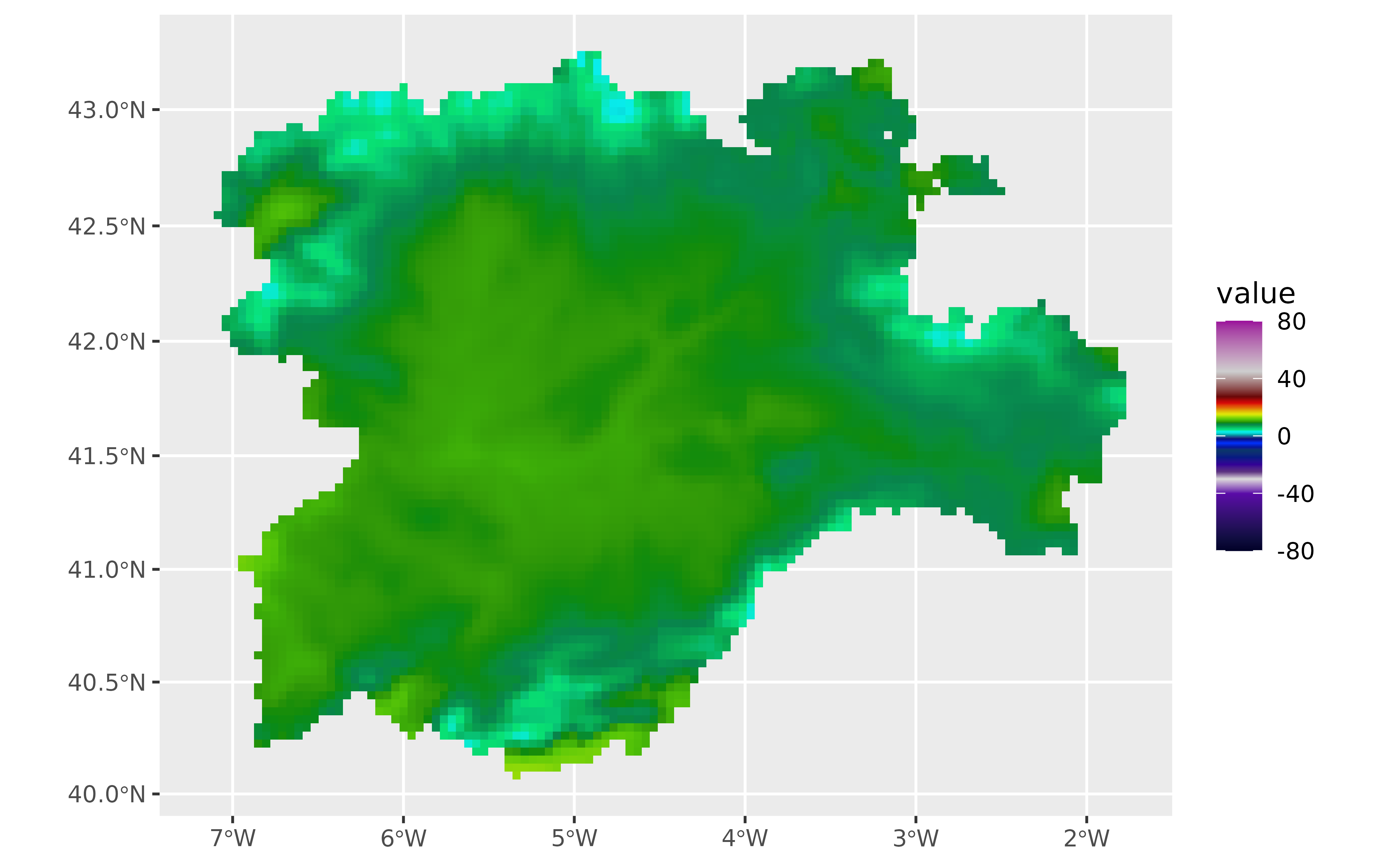# Display facets
ggplot() +
geom_spatraster(data = temp_rast) +
facet_wrap(~lyr, ncol = 2) +
scale_fill_hypso_b()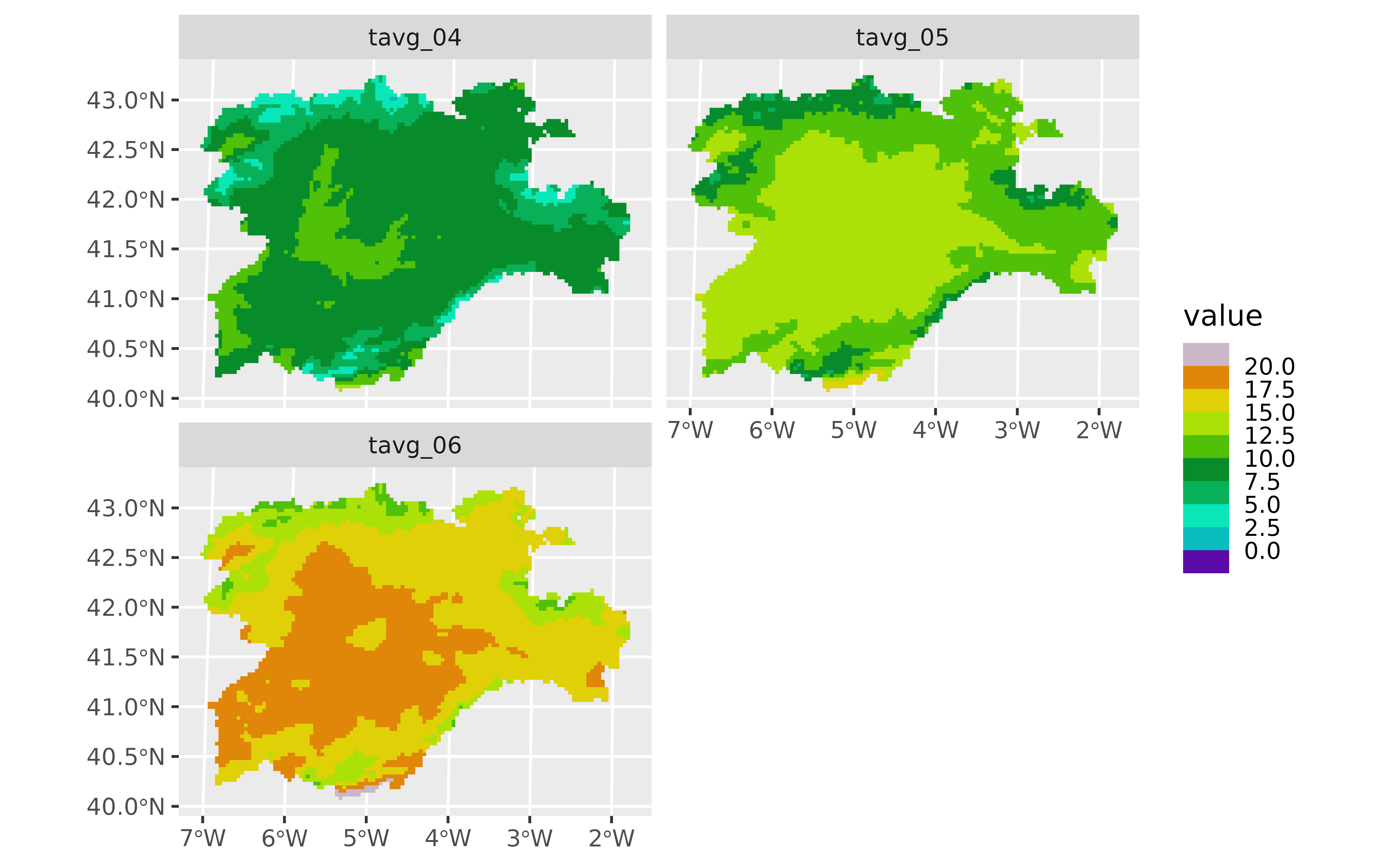# Non spatial rasters

no_crs <- rast(crs = NA, extent = c(0, 100, 0, 100), nlyr = 1)
values(no_crs) <- seq_len(ncell(no_crs))

ggplot() +
geom_spatraster(data = no_crs)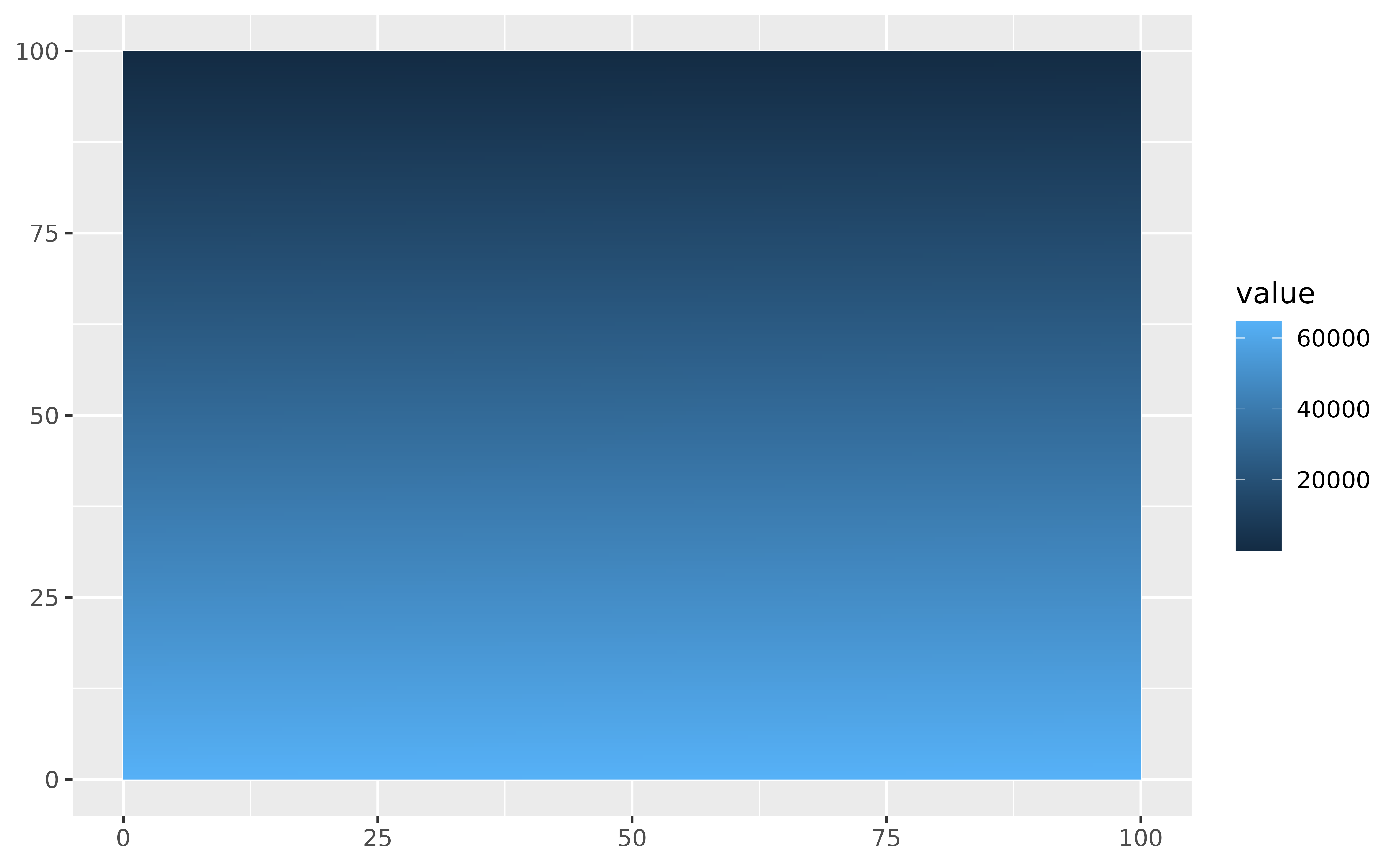# Downsample

ggplot() +
geom_spatraster(data = no_crs, maxcell = 25)
#> SpatRaster resampled to ncells = 32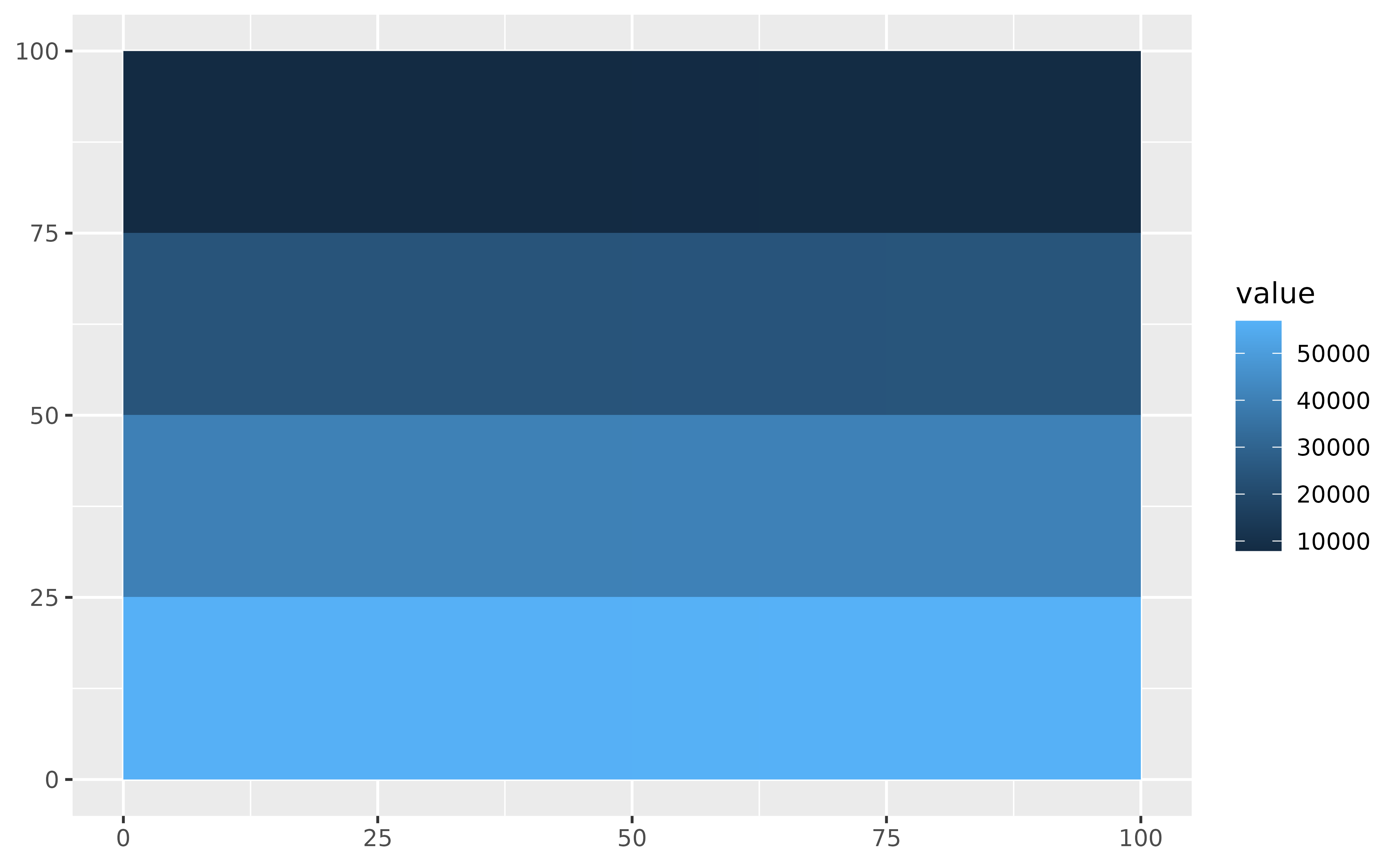# }

# \donttest{
# Using stat_spatraster
# Default
ggplot() +
stat_spatraster(data = temp_rast) +
facet_wrap(~lyr)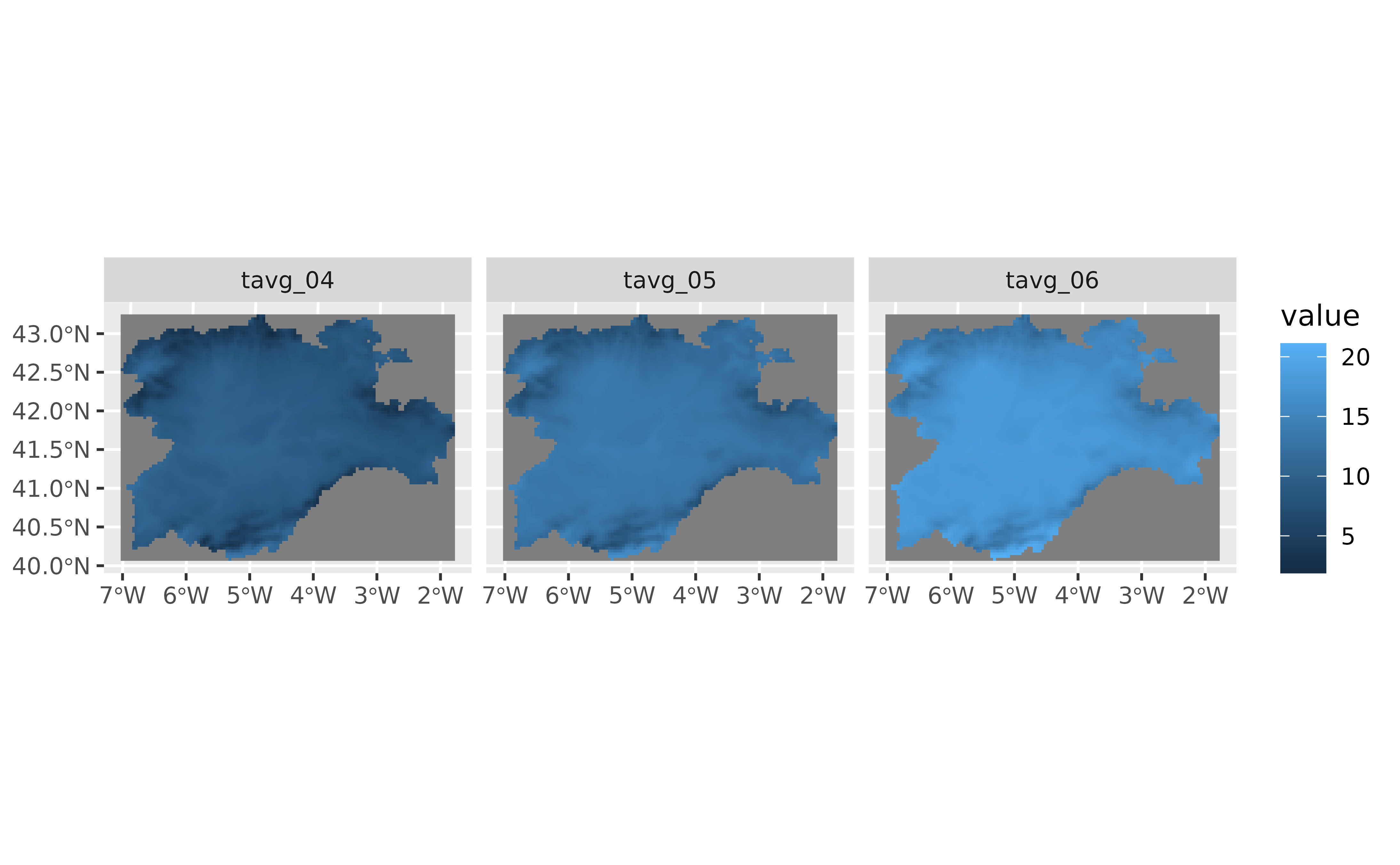# Using points
ggplot() +
stat_spatraster(
data = temp_rast,
aes(color = after_stat(value)),
geom = "point", maxcell = 250
) +
scale_colour_viridis_c(na.value = NA) +
facet_wrap(~lyr)
#> SpatRaster resampled to ncells = 266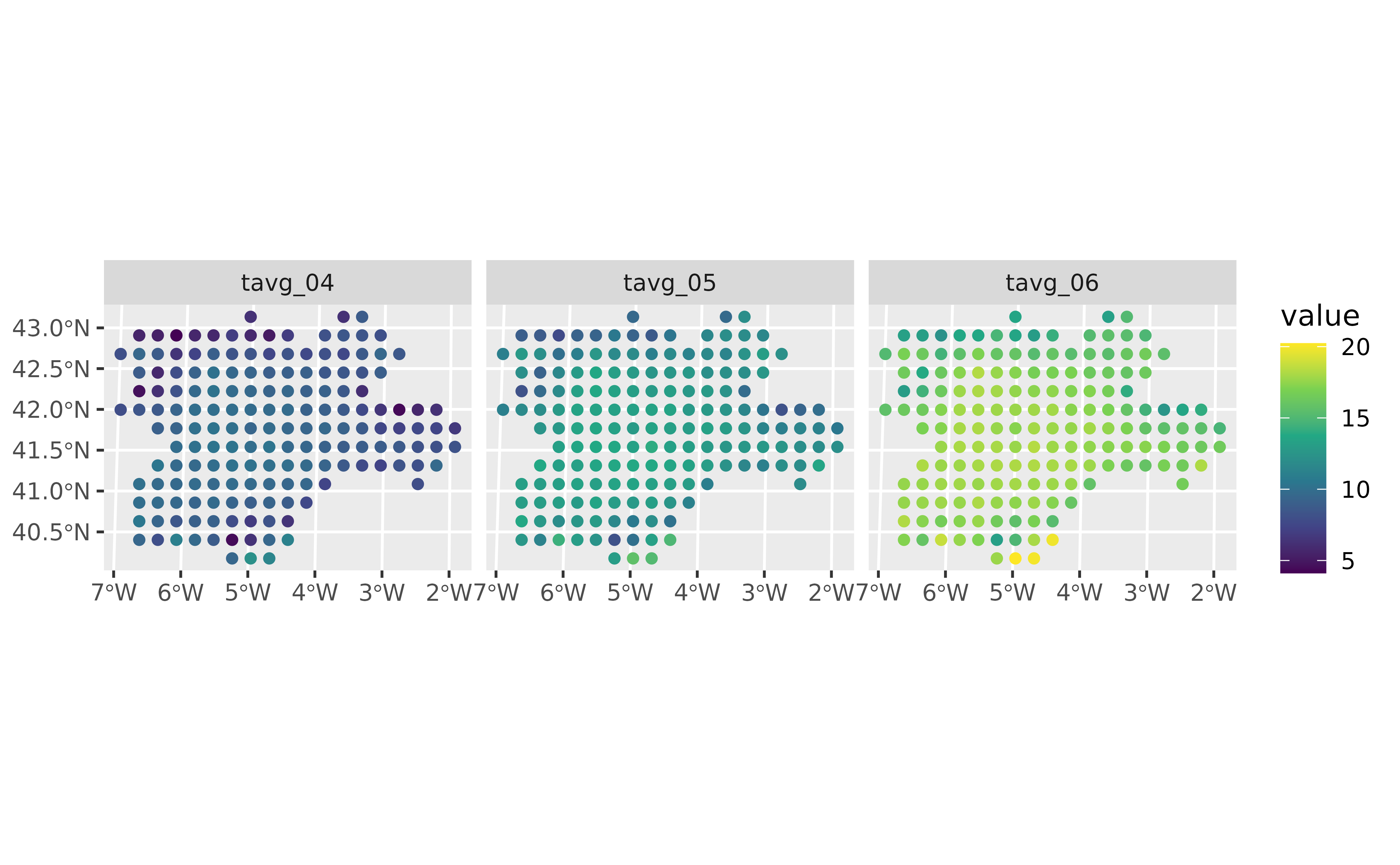# Using points and labels

r_single <- temp_rast %>% select(1)

ggplot() +
stat_spatraster(
data = r_single,
aes(color = after_stat(value)),
geom = "point",
maxcell = 2000
) +
stat_spatraster(
data = r_single,
aes(label = after_stat(round(value, 2))),
geom = "label",
alpha = 0.85,
maxcell = 20
) +
scale_colour_viridis_c(na.value = NA)
#> SpatRaster resampled to ncells = 2067
#> SpatRaster resampled to ncells = 24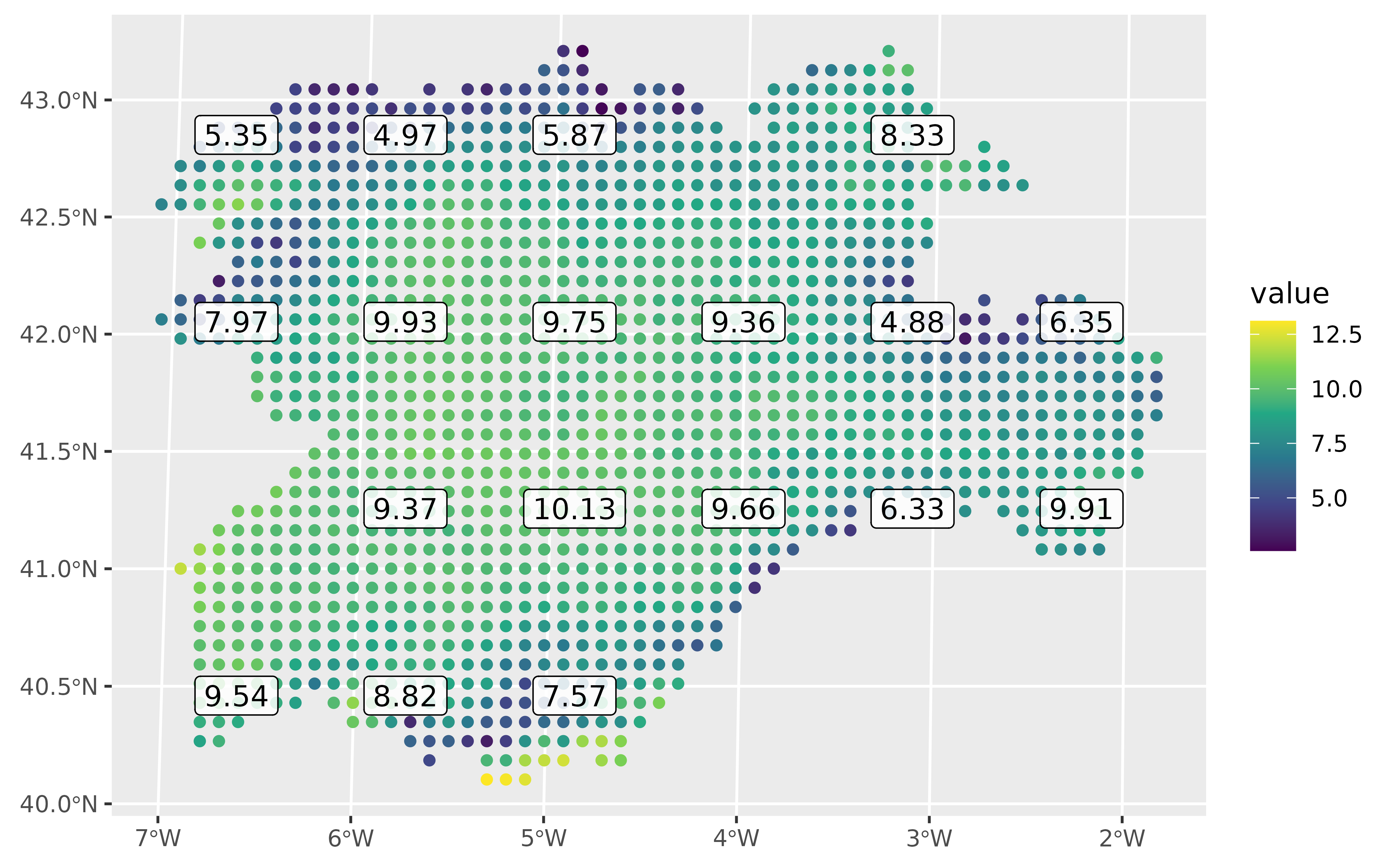# }